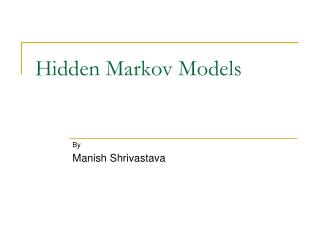DownloadDownload PresentationHidden Markov Models

# Hidden Markov Models

Download Presentation## Hidden Markov Models

- - - - - - - - - - - - - - - - - - - - - - - - - - - E N D - - - - - - - - - - - - - - - - - - - - - - - - - - -
##### Presentation Transcript

1. Hidden Markov Models By Manish Shrivastava

2. A Simple Process b S1 b a S2 a A simple automata

3. A Slightly Complicated Process A colored ball choosing example : Urn 1 # of Red = 100 # of Green = 0 # of Blue = 0 Urn 3 # of Red = 0 # of Green = 0 # of Blue = 100 Urn 2 # of Red = 0 # of Green = 100 # of Blue = 0 Probability of transition to another Urn after picking a ball:

4. A Slightly Complicated Process contd. Given : Observation : RRGGBRGR State Sequence : ?? Easily Computable.

5. Markov Processes • Properties • Limited Horizon :Given previous n states, a state i, is independent of preceding 0…i-n+1 states. • P(Xt=i|Xt-1, Xt-2 ,…X0) = P(Xt=i|Xt-1, Xt-2… Xt-n) • Time invariance : • P(Xt=i|Xt-1=j) = P(X1=i|X0=j) = P(Xn=i|X0-1=j)

6. A (Slightly Complicated) Markov Process A colored ball choosing example : Urn 1 # of Red = 100 # of Green = 0 # of Blue = 0 Urn 3 # of Red = 0 # of Green = 0 # of Blue = 100 Urn 2 # of Red = 0 # of Green = 100 # of Blue = 0 Probability of transition to another Urn after picking a ball:

7. Markov Process • Visible Markov Model • Given the observation, one can easily follow the state sequence traversed 1 2 3 P(1|3) P(3|1)

8. Hidden Markov Model A colored ball choosing example : Urn 1 # of Red = 30 # of Green = 50 # of Blue = 20 Urn 3 # of Red =60 # of Green =10 # of Blue = 30 Urn 2 # of Red = 10 # of Green = 40 # of Blue = 50 Probability of transition to another Urn after picking a ball:

9. Hidden Markov Model Given : and Observation : RRGGBRGR State Sequence : ?? Not so Easily Computable.

10. Hidden Markov Model • Set of states : S • Output Alphabet : V • Transition Probabilities : A = {aij} • Emission Probabilities : B = {bj(ok)} • Initial State Probabilities : π

11. Hidden Markov Model • Here : • S = {U1, U2, U3} • V = { R,G,B} • For observation: • O ={o1… on} • And State sequence • Q ={q1… qn} • π is A = B=

12. Three Basic Problems of HMM • Given Observation Sequence O ={o1… on} • Efficiently estimate • Given Observation Sequence O ={o1… on} • Get best Q ={q1… qn} • How to adjust to best maximize

13. Questions?

14. References • Lawrence R. Rabiner, A Tutorial on Hidden Markov Models and Selected Applications in Speech Recognition. Proceedings of the IEEE, 77 (2), p. 257–286, February 1989. • Chris Manning and Hinrich Schütze, Chapter 9: Markov Models,Foundations of Statistical Natural Language Processing, MIT Press. Cambridge, MA: May 1999